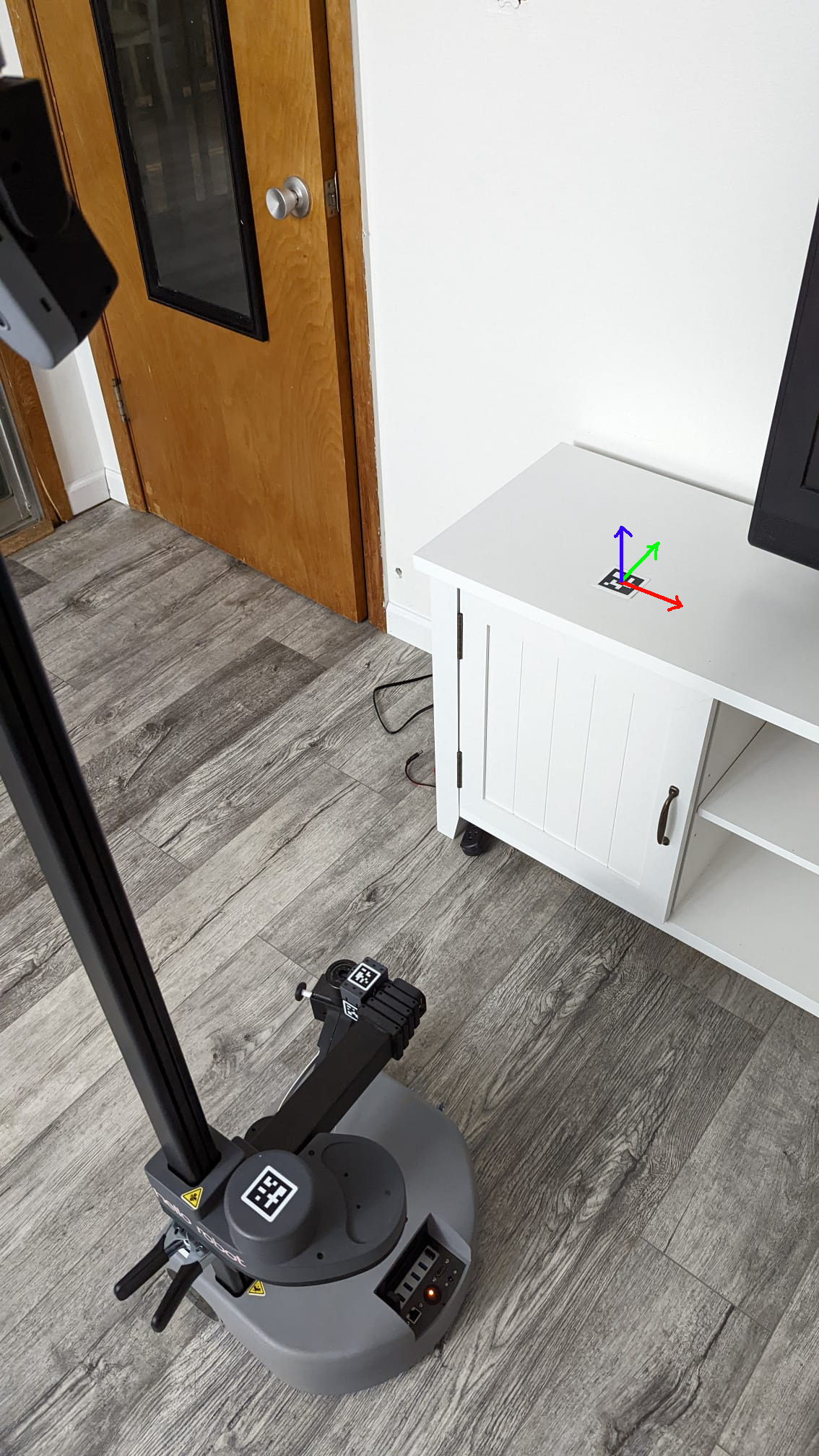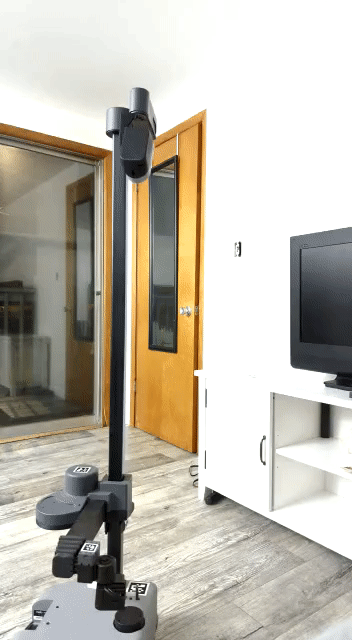# Align to ArUco

ArUco markers are a type of fiducials that are used extensively in robotics for identification and pose estimation. In this tutorial we will learn how to identify ArUco markers with the ArUco detection node and enable Stretch to navigate and align itself with respect to the marker.

## ArUco Detection

Stretch uses the OpenCV ArUco detection library and is configured to identify a specific set of ArUco markers belonging to the 6x6, 250 dictionary. To understand why this is important, please refer to this handy guide provided by OpenCV.

Stretch comes preconfigured to identify ArUco markers. The ROS node that enables this is the detect_aruco_markers node in the stretch_core package. Thanks to this node, identifying and estimating the pose of a marker is as easy as pointing the camera at the marker and running the detection node. It is also possible and quite convenient to visualize the detections with RViz.

## Computing Transformations

If you have not already done so, now might be a good time to review the tf listener tutorial. Go on, we can wait… Now that we know how to program stretch to return the transform between known reference frames, we can use this knowledge to compute the transform between the detected marker and the robot base_link. From its current pose, for Stretch to align itself in front of the marker, we need to command it to reach there. But even before that, we need to program Stretch to know the goal pose. We define the goal pose to be 0.5 metre outward from the marker in the marker negative y-axis (Green axis). This is easier to visualize through the figure below.By monitoring the /aruco/marker_array and /aruco/axes topics, we can visualize the markers in RViz. The detection node also publishes the tf pose of the detected markers. This can be visualized by using the TF plugin and selecting the detected marker to inspect the pose. Next, we will use exactly that to compute the transform between the detected marker and the base_link of the robot.

Now, we can compute the transformation from the robot base_link frame to the goal pose and pass this as an SE2 pose to the mobile base.

Since we want Stretch to stop at a fixed distance with respect to the marker, we define a 0.5m offset in the marker y-axis where Stretch would come to a stop. At the same time, we also want Stretch to align its orientation to point its arm towards the marker so as to make the subsequent manipulation tasks easier to accomplish. This would result in the end pose of the base_link as shown in the above figure. Sweet! The next task is to generate a simple motion plan for the mobile base to reach this end pose. We do this in three steps: 1. Turn theta degrees towards the goal position. This would be the angle formed between the robot x-axis and the line connecting the start and the goal positions. 2. Travel straight to the goal position. This would be the euclidean distance between the start and the goal positions. 3. Turn phi degrees to attain the goal orientation. This would be the correction angle necessary to align the robot y-axis with the marker x-axis.

Luckily, we know how to command Stretch to execute a trajectory using the joint trajectory server. If you are just starting, have a look at the Follow Joint Trajectory Commands tutorial to know how to command Stretch using the Joint trajectory Server.

Warning

Since we won't be using the arm for this demo, it's safer to stow Stretch's arm in.

``````stretch_robot_stow.py
``````

## See It In Action

First, we need to point the camera towards the marker. To do this, you could use the keyboard teleop node. To do this, run:

``````ros2 launch stretch_core keyboard_teleop.launch.py
``````

When you are ready, execute the following command:

``````ros2 launch stretch_core align_to_aruco.launch.py
``````## Code Breakdown

Let's jump into the code to see how things work under the hood. Follow along here to have a look at the entire script.

We make use of two separate Python classes for this demo. The FrameListener class is derived from the Node class and is the place where we compute the TF transformations. For an explantion of this class, you can refer to the TF listener tutorial.

``````class FrameListener(Node):
``````

The AlignToAruco class is where we command Stretch to the pose goal. This class is derived from the FrameListener class so that they can both share the node instance.

``````class AlignToAruco(FrameListener):
``````

The constructor initializes the Joint trajectory action client. It also initializes the attribute called offset that determines the end distance between the marker and the robot.

``````    def __init__(self, node, offset=0.75):
self.trans_base = TransformStamped()
self.trans_camera = TransformStamped()
self.joint_state = JointState()
self.offset = offset
self.node = node

self.trajectory_client = ActionClient(self.node, FollowJointTrajectory, '/stretch_controller/follow_joint_trajectory')
server_reached = self.trajectory_client.wait_for_server(timeout_sec=60.0)
if not server_reached:
self.node.get_logger().error('Unable to connect to arm action server. Timeout exceeded.')
sys.exit()
``````

The joint_states_callback is the callback method that receives the most recent joint state messages published on the /stretch/joint_states topic.

``````    def joint_states_callback(self, joint_state):
self.joint_state = joint_state
``````

The copute_difference() method is where we call the get_transform() method from the FrameListener class to compute the difference between the base_link and base_right frame with an offset of 0.5 m in the negative y-axis.

``````    def compute_difference(self):
self.trans_base, self.trans_camera = self.node.get_transforms()
``````

To compute the (x, y) coordinates of the SE2 pose goal, we compute the transformation here.

``````        R = quaternion_matrix((x, y, z, w))
P_dash = np.array([, [-self.offset], , ])
P = np.array([[self.trans_base.transform.translation.x], [self.trans_base.transform.translation.y], , ])

X = np.matmul(R, P_dash)
P_base = X + P

base_position_x = P_base[0, 0]
base_position_y = P_base[1, 0]
``````

From this, it is relatively straightforward to compute the angle phi and the euclidean distance dist. We then compute the angle z_rot_base to perform the last angle correction.

``````        phi = atan2(base_position_y, base_position_x)

dist = sqrt(pow(base_position_x, 2) + pow(base_position_y, 2))

x_rot_base, y_rot_base, z_rot_base = euler_from_quaternion([x, y, z, w])
z_rot_base = -phi + z_rot_base + 3.14159
``````

The align_to_marker() method is where we command Stretch to the pose goal in three steps using the Joint Trajectory action server. For an explanation on how to form the trajectory goal, you can refer to the Follow Joint Trajectory Commands tutorial.

``````    def align_to_marker(self):
``````

If you want to work with a different ArUco marker than the one we used in this tutorial, you can do so by changing line 44 in the code to the one you wish to detect. Also, don't forget to add the marker in the stretch_marker_dict.yaml ArUco marker dictionary.